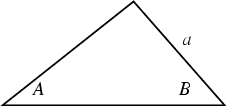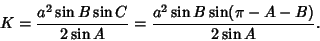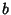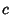## AAS TheoremSpecifying two anglesandand a sideuniquely determines a Triangle with Area(1)

The third angle is given by(2)

since the sum of angles of a Triangle is 180° (Radians). Solving the Law of Sines(3)

forgives(4)

Finally,(5)(6)

See also AAA Theorem, ASA Theorem, ASS Theorem, SAS Theorem, SSS Theorem, Triangle